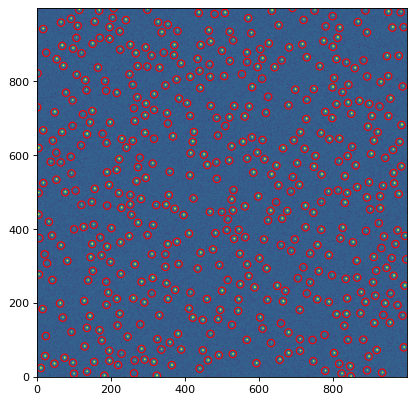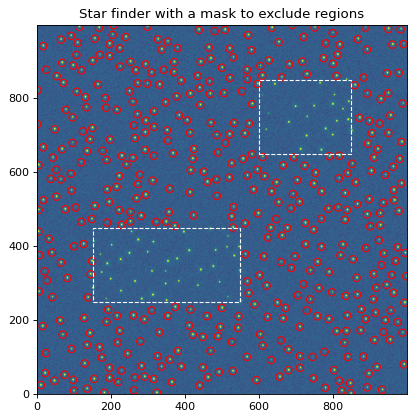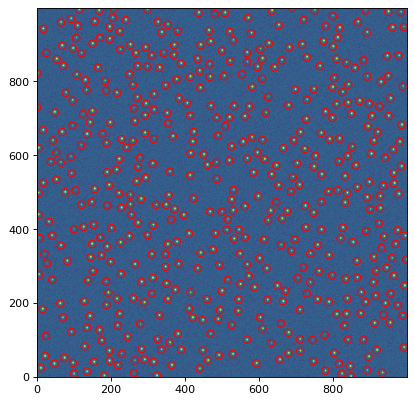# Source Detection (`photutils.detection`)¶

## Introduction¶

One generally needs to identify astronomical sources in their data before they can perform photometry or morphological measurements. Photutils provides two functions designed specifically to detect point-like (stellar) sources in an astronomical image. Photutils also provides a function to identify local peaks in an image that are above a specified threshold value.

For general-use source detection and extraction of both point-like and extended sources, please see Image Segmentation.

## Detecting Stars¶

Photutils includes two widely-used tools that are used to detect stars in an image, DAOFIND and IRAF’s starfind.

`DAOStarFinder` is a class that provides an implementation of the DAOFIND algorithm (Stetson 1987, PASP 99, 191). It searches images for local density maxima that have a peak amplitude greater than a specified threshold (the threshold is applied to a convolved image) and have a size and shape similar to a defined 2D Gaussian kernel. `DAOStarFinder` also provides an estimate of the objects’ roundness and sharpness, whose lower and upper bounds can be specified.

`IRAFStarFinder` is a class that implements IRAF’s starfind algorithm. It is fundamentally similar to `DAOStarFinder`, but `DAOStarFinder` can use an elliptical Gaussian kernel. One other difference in `IRAFStarFinder` is that it calculates the objects’ centroid, roundness, and sharpness using image moments.

As an example, let’s load an image from the bundled datasets and select a subset of the image. We will estimate the background and background noise using sigma-clipped statistics:

```>>> from astropy.stats import sigma_clipped_stats
>>> data = hdu.data[0:401, 0:401]
>>> mean, median, std = sigma_clipped_stats(data, sigma=3.0)
>>> print((mean, median, std))
(3668.09661145823, 3649.0, 204.41388592022315)
```

Now we will subtract the background and use an instance of `DAOStarFinder` to find the stars in the image that have FWHMs of around 3 pixels and have peaks approximately 5-sigma above the background. Running this class on the data yields an astropy `Table` containing the results of the star finder:

```>>> from photutils.detection import DAOStarFinder
>>> daofind = DAOStarFinder(fwhm=3.0, threshold=5.*std)
>>> sources = daofind(data - median)
>>> for col in sources.colnames:
...     sources[col].info.format = '%.8g'  # for consistent table output
>>> print(sources)
id xcentroid ycentroid sharpness  ... sky peak    flux       mag
--- --------- --------- ---------- ... --- ---- --------- ------------
1 144.24757 6.3797904 0.58156257 ...   0 6903 5.6976747   -1.8892441
2 208.66907 6.8205805 0.48348966 ...   0 7896 6.7186388   -2.0682032
3 216.92614 6.5775933 0.69359525 ...   0 2195 1.6662764  -0.55436758
4 351.62519 8.5459013 0.48577834 ...   0 6977 5.8970385   -1.9265849
5 377.51991 12.065501 0.52038488 ...   0 1260 1.1178252  -0.12093477
...       ...       ...        ... ... ...  ...       ...          ...
282 267.90091 398.61991 0.27117231 ...   0 9299 5.4379278   -1.8385836
283 271.46959 398.91242 0.36738752 ...   0 8028 5.0693475   -1.7623802
284 299.05003 398.78469 0.25895667 ...   0 9072 5.5584641    -1.862387
285 299.99359 398.76661 0.29412474 ...   0 9253 5.3233471    -1.815462
286 360.44533 399.52381 0.37315624 ...   0 8079 6.9203438   -2.1003192
Length = 286 rows
```

Let’s plot the image and mark the location of detected sources:

```>>> import numpy as np
>>> import matplotlib.pyplot as plt
>>> from astropy.visualization import SqrtStretch
>>> from astropy.visualization.mpl_normalize import ImageNormalize
>>> from photutils.aperture import CircularAperture
>>> positions = np.transpose((sources['xcentroid'], sources['ycentroid']))
>>> apertures = CircularAperture(positions, r=4.)
>>> norm = ImageNormalize(stretch=SqrtStretch())
>>> plt.imshow(data, cmap='Greys', origin='lower', norm=norm,
...            interpolation='nearest')
>>> apertures.plot(color='blue', lw=1.5, alpha=0.5)
```Regions of the input image can be masked by using the `mask` keyword with the `DAOStarFinder` or `IRAFStarFinder` instance. This simple examples uses `DAOStarFinder` and masks two rectangular regions. No sources will be detected in the masked regions:

```>>> from photutils.detection import DAOStarFinder
>>> daofind = DAOStarFinder(fwhm=3.0, threshold=5.*std)
>>> for col in sources.colnames:
>>>     sources[col].info.format = '%.8g'  # for consistent table output
>>> print(sources)
```## Local Peak Detection¶

Photutils also includes a `find_peaks()` function to find local peaks in an image that are above a specified threshold value. Peaks are the local maxima above a specified threshold that are separated by a specified minimum number of pixels.

By default, the returned pixel coordinates are always integer-valued (i.e., no centroiding is performed, only the peak pixel is identified). However, a centroiding function can be input via the `centroid_func` keyword to `find_peaks()` to compute centroid coordinates with subpixel precision.

`find_peaks()` supports a number of additional options, including searching for peaks only within a specified footprint. Please see the `find_peaks()` documentation for more options.

As a simple example, let’s find the local peaks in an image that are 5 sigma above the background and a separated by at least 5 pixels:

```>>> from astropy.stats import sigma_clipped_stats
>>> from photutils.datasets import make_100gaussians_image
>>> from photutils.detection import find_peaks
>>> data = make_100gaussians_image()
>>> mean, median, std = sigma_clipped_stats(data, sigma=3.0)
>>> threshold = median + (5. * std)
>>> tbl = find_peaks(data, threshold, box_size=11)
>>> tbl['peak_value'].info.format = '%.8g'  # for consistent table output
>>> print(tbl[:10])  # print only the first 10 peaks
x_peak y_peak peak_value
------ ------ ----------
233      0  27.477852
493      6  20.404769
207     11  24.075798
258     12  17.395025
366     12  18.729726
289     22  35.853276
380     29  19.261986
442     31  30.239994
359     36  19.771626
471     38   25.45583
```

And let’s plot the location of the detected peaks in the image:

```>>> import numpy as np
>>> import matplotlib.pyplot as plt
>>> from astropy.visualization import simple_norm
>>> from astropy.visualization.mpl_normalize import ImageNormalize
>>> from photutils.aperture import CircularAperture
>>> positions = np.transpose((tbl['x_peak'], tbl['y_peak']))
>>> apertures = CircularAperture(positions, r=5.)
>>> norm = simple_norm(data, 'sqrt', percent=99.9)
>>> plt.imshow(data, cmap='Greys_r', origin='lower', norm=norm,
...            interpolation='nearest')
>>> apertures.plot(color='#0547f9', lw=1.5)
>>> plt.xlim(0, data.shape-1)
>>> plt.ylim(0, data.shape-1)
```## Reference/API¶

This subpackage contains tools for detecting sources in an astronomical image.

### Functions¶

 `find_peaks`(data, threshold[, box_size, …]) Find local peaks in an image that are above above a specified threshold value.

### Classes¶

 `DAOStarFinder`(threshold, fwhm[, ratio, …]) Detect stars in an image using the DAOFIND (Stetson 1987) algorithm. `IRAFStarFinder`(threshold, fwhm[, …]) Detect stars in an image using IRAF’s “starfind” algorithm. `StarFinder`(threshold, kernel[, …]) Detect stars in an image using a user-defined kernel. Abstract base class for star finders.